﻿ 应用指数函数方法求解KdV型方程 Application of Exp-Function Method to Solve KdV-Type Equation

Advances in Applied Mathematics
Vol.04 No.04(2015), Article ID:16435,7 pages
10.12677/AAM.2015.44046

Application of Exp-Function Method to Solve KdV-Type Equation

Sai Zhang, Guofang Li, Ning Wang

College of Sciences, North China University of Technology, Beijing

Received: Nov. 5th, 2015; accepted: Nov. 20th, 2015; published: Nov. 27th, 2015

Copyright © 2015 by authors and Hans Publishers Inc.

This work is licensed under the Creative Commons Attribution International License (CC BY).

http://creativecommons.org/licenses/by/4.0/ABSTRACT

Exp-function method is an effective way to construct exact solutions of partial differential equations in mathematics and physics. This paper applies Exp-function method to obtain the new exact solutions of KdV-type equation, and depicts the figures of the solutions respectively in order to better understand the properties of the solutions.

Keywords:Exp-Function Method, KdV-Type Equation, Exact Solution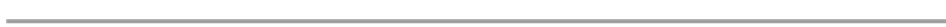1. 引言

1895年，荷兰的特韦格(Korteweg)和德弗里斯(de Vries)在研究浅水中小振幅长波运动时，共同得出了一种单向运动浅水波偏微分方程，即KdV Equation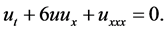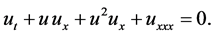(1.1)

2. 方法概述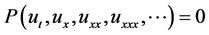(2.1)

1) 假设方程(2.1)具有行波解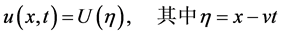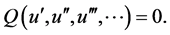(2.2)

2) 假设(2.2)的方程具有如下形式：(2.3)

3) 将(2.3)代入到(2.2)中，然后平衡(2.2)中的非线性项和最高阶导数项的最高次数可以得到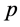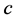的关系。同理，平衡非线性项和最高阶导数项的最低次数可以得到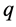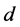的关系。

4) 对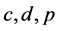赋特殊值，并将(2.3)代入(2.2)中可以得到关于的方程组，之后，令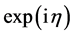的系数为零，可得到一系列关于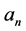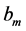的代数方程，求解这些代数方程并将结果代入(2.3)中，即可求得(2.1)的解.

3. 方程(1.1)求解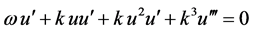(3.1)(3.2)(3.3)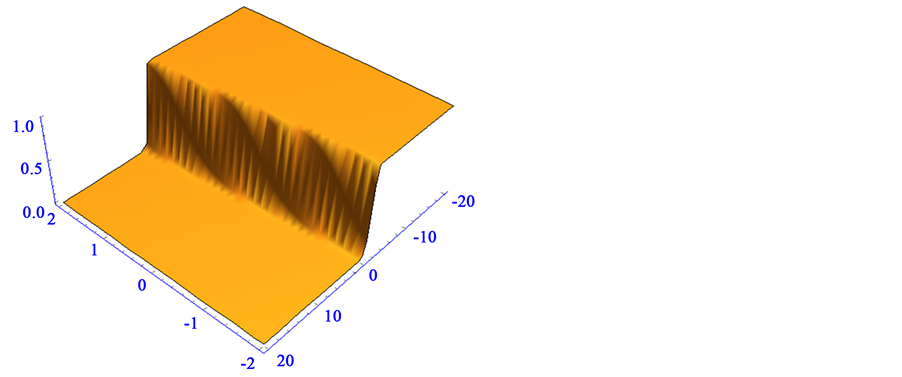Figure 1. Solution for Case 1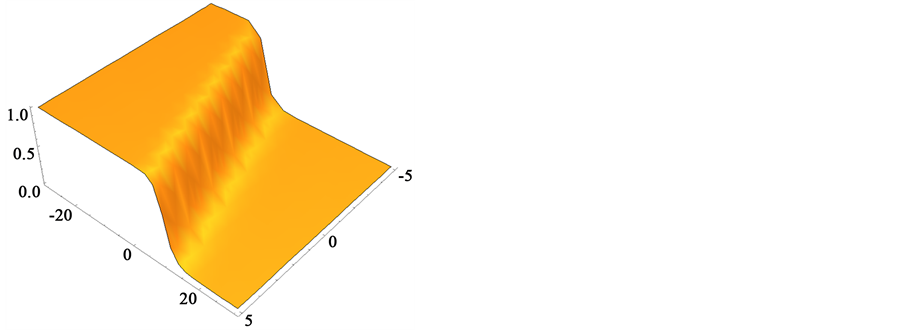Figure 2. Solution for Case 2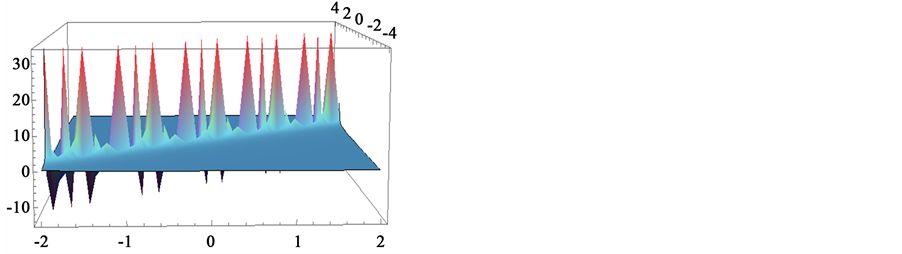Figure 3. Solution for Case 3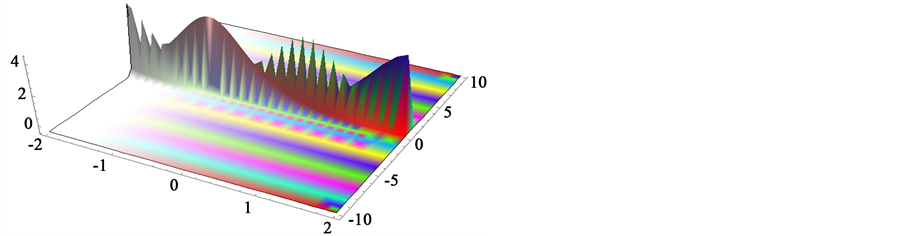Figure 4. Solution for Case 4Figure 5. Solution for Case 1.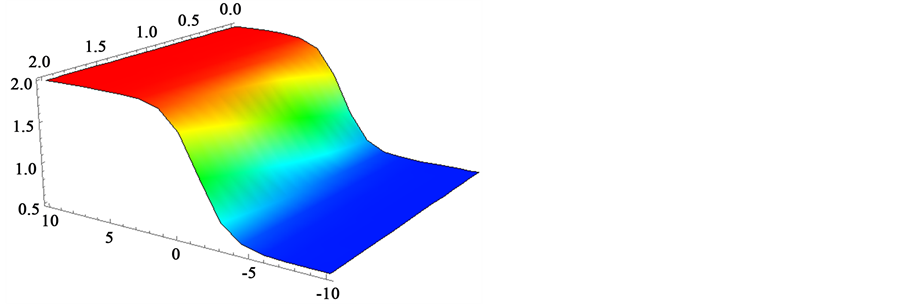Figure 6. Solution for Case 6.

4. 总结

Application of Exp-Function Method to Solve KdV-Type Equation[J]. 应用数学进展, 2015, 04(04): 369-375. http://dx.doi.org/10.12677/AAM.2015.44046

1. 1. Ablowitz, M.J. and Clarkson, P.A. (1991) Solitons, Nonlinear Evolution Equations and Inverse Scattering. Cambridge University Press, New York. http://dx.doi.org/10.1017/CBO9780511623998

2. 2. 闫振亚. 复杂非线性波的构造性理论及其应用[M]. 北京: 科学出版社, 2007.

3. 3. 范恩贵, 张鸿庆. 非线性孤子方程的齐次平衡法[J]. 物理学报, 1998(47): 333-361.

4. 4. 李志斌, 张善卿. 非线性波动方程准确孤立波解的符号计算[J]. 数学物理学报, 1997(17): 81-89.

5. 5. 楼森岳, 唐晓艳. 非线性数学物理方程[M]. 北京: 科学出版社, 2006.

6. 6. He, J.-H. and Wu, X.-H. (2006) Exp-Function Method for Nonlinear Wave Equations. Chaos, Solitons and Fractals, 30, 700-708. http://dx.doi.org/10.1016/j.chaos.2006.03.020

7. 7. Bluman, G.W. and Anco, S.C. (2002) Symmetry and Integration Methods for Differential Equations. Springer, New York.

8. 8. Dey, B. (1985) Domain Wall Solution of KdV like Eq-uation with Higher Order Nonlinearity. Journal of Physics A: Mathematical and General, 19, L9-L12. http://dx.doi.org/10.1088/0305-4470/19/1/003

9. 9. Zhang, W.G., Chang, Q.S. and Jiang, B.G. (2002) Explicit Exact Solitary-Wave Solutions for the Compound KdV- Type and Compound KdV-Burgers-Type Equations with Nonlinear Terms of Any Order. Chaos, Solitons and Fractals, 13, 311-319. http://dx.doi.org/10.1016/S0960-0779(00)00272-1